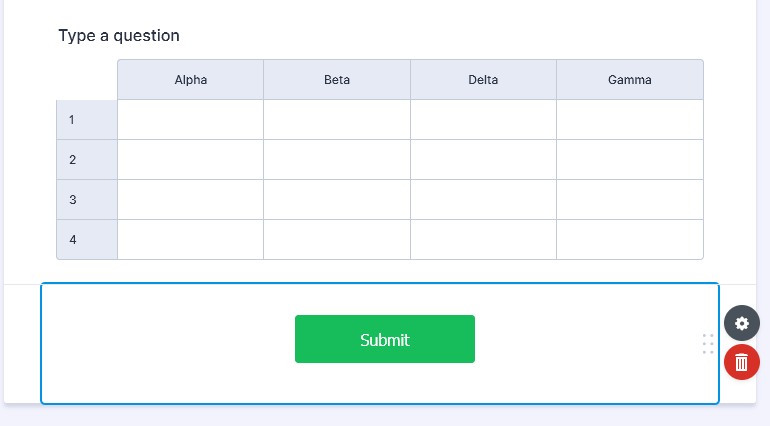Use InputTable - Calculate in a column

•MonarchForms
Asked on November 12, 2021 at 08:18 AM

When using the InputTable as a method is there a way to use formulas or calculations in on column?

In this image below I would have row 1 calculate in Row 1 of the Gamma field:•ashwin_d
Answered on November 12, 2021 at 09:24 AM

Yes, it is possible to perform calculation in input table field. You may like to take a look at the following guide, which should help you: https://www.jotform.com/help/343-how-to-perform-form-calculation-in-the-input-table-field/

You can also add conditions in form to calculate the values of input table and the following guide should help you: https://www.jotform.com/help/414-calculate-values-in-the-input-table-through-condition/

Hope this helps.

Do try it out and get back to us if you have any questions.